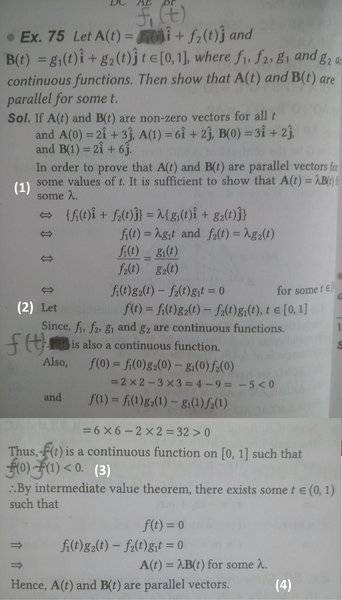# Parallelism of Time-varying Vectors

baldbrain

## Homework Statement

This is a solved problem, but I haven't understood a few things.
I've marked out sections of the solution in white for convenience. The markings are positioned where that particular section ends.In part (1), how did they just assume
f1(0) = 2, f2(0) = 3, g1(0) = 3, g2(0) = 2
f1(1) = 6, f2(1) = 2, g1(1) = 2, g2(1) = 6

And, in part (4), what is this 'intermediate value theorem' that they've used?
We've just done the basics on vectors, so I have no idea where this 'intermediate value theorem' came from....
Then, in part (2) they say we have to prove that
f1(t).g2(t) - f2(t).g1(t) = 0
And in part (4), they just implied the same thing from out of nowhere & voila! The problem's over!

#### Attachments

Gold Member
Your objections are valid. These values seem to come out of nowhere.

The intermediate value theorem simply says that if a continuous function has two values, then it must also have every value in between the two.

•baldbrain
baldbrain
The intermediate value theorem simply says that if a continuous function has two values, then it must also have every value in between the two.
That's so obvious (assuming the function is defined on R). We've done this as a deduction of continuity, not as a separate theorem.

baldbrain
These values have a pattern...
2 3 3 2
6 2 2 6

Ok professor